# Angle Sum Property of a Quadrilateral

• Last Updated : 07 Nov, 2021

Quadrilaterals are encountered everywhere in life, every square rectangle, any shape with four sides is a quadrilateral. We know, three non-collinear points make a triangle. Similarly, four non-collinear points take up a shape that is called a quadrilateral. It has four sides, four angles, and four vertices.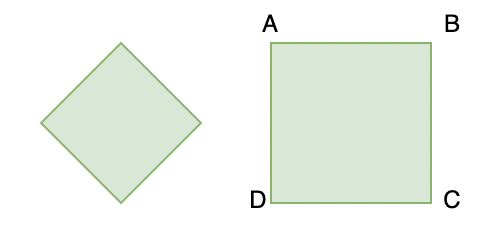Both the figures above are examples of quadrilaterals. ABCD is a quadrilateral. AB, BC, CD, and DA are four sides of the quadrilateral. A, B, C, and D are four vertices, and ∠A, ∠B, ∠C, and ∠D are the angles of this quadrilateral.

### Some Important Terminology

Let’s look at some terms and conventions related to quadrilaterals.

Opposite Sides: Two Sides of the quadrilateral are called opposite sides if they have no common vertex.

For example: In the figure given above look at the quad ABCD. Here, AB and CD are opposite sides. Similarly, AD and BC are opposite sides.

Opposite Angles: Two angles of a quadrilateral are opposite if they don’t have any common arm.

For example: In the figure ABCD again, angle A and angle C don’t have any common arm. Thus, they can be considered as opposite angles. Similarly, angles B and D are also opposite angles.

Adjacent Sides: Two sides are called adjacent if sides have a common vertex.

For example: AB and AD have common vertex “A”. So, they are called adjacent sides. Similarly, AB, BC; BC, CD and AD, DC are adjacent sides.

Adjacent Angles: Two angles, if they have a common arm are called adjacent angles.

For example: ∠A, ∠B are adjacent angles.

Question: List the pair of opposite sides and adjacent angles from the quadrilateral given below.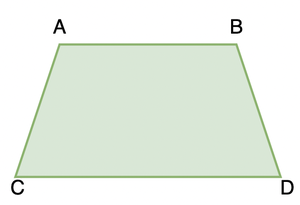Pair of opposite sides are the sides which don’t have any common vertices.

So, in this case (AB, CD) and (AC, BD) are two pairs of opposite sides.

Similarly, going by the definition given above. Pair of adjacent sides are,

(AC, AB); (AB, BD); (BD, DC); (CD, AC)

Quadrilaterals can be classified into five types:

1. Parallelogram: It is quadrilateral which has its opposite sides parallel and congruent to each other. The opposite angles are also equal.
2. Rectangle: It is a quadrilateral that has its opposite sides equal and all the angles are at the right angle(90°).
3. Square: It is a quadrilateral that has all its sides of equal length and all the angles are at the right angle(90°).
4. Rhombus: It is a parallelogram that has all of its sides of equal length.
5. Trapezium: It has one pair of parallel sides. Its sides may or may not be of equal length.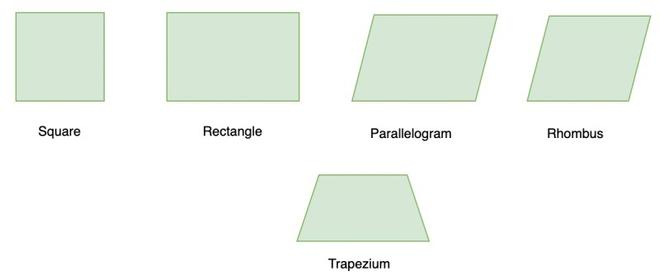### Angle Sum Property

This property states that the sum of all angles of a quadrilateral is 360°. Let’s prove this.

Theorem: The sum of all the four angles of a quadrilateral is 360°.

Proof: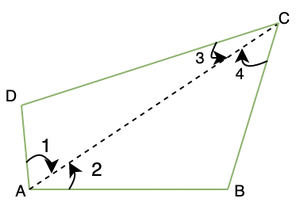Join AC.

Now notice,

∠1 + ∠2 = ∠A

∠3 + ∠4 = ∠C

Therefore, from triangle ABC

∠4 + ∠2 + ∠B = 180o

∠3 + ∠1 + ∠D = 180o

∠4 + ∠2 + ∠B + ∠3 + ∠1 + ∠D = 360o

⇒ (∠1 + ∠2) + (∠3+ ∠4) + ∠B + ∠D = 360o

⇒ ∠A + ∠C + ∠B + ∠D = 360o

Thus, this proves that sum of all interior angles of a quadrilateral is 360°.

### Sample Problems

Question 1: The angles of a quadrilateral are 60°, 90°, 90°. Find the fourth remaining angle.

Solution:

We know from the angle sum property that the sum of the angles of a quadrilateral are 360o

Let the fourth angle be denoted by “x”.

So,

60° + 90°+ 90° + x = 360°

⇒ 180° + 60° + x = 360°

⇒ 240° + x = 360°

⇒ x = 120°

Question 2: The angles of a quadrilateral are given to be (3x)°, (3x + 30)°, (6x + 60)°, 90°. Find the value of all the angles of quadrilaterals.

Solution:

We know, sum of all the angles of quadrilateral are 360°.

3x + (3x + 30) + (6x + 60) + 90 = 360

⇒ (3x + 3x + 6x) + (30 + 60 + 90) = 360

⇒ (12x) + (180) = 360

⇒ 12x = 360 – 180

⇒ 12x = 180

⇒ x = 15°

Thus, the angles are 45°, 75°, 150° and 90°

Question 3: If the angles of a quadrilateral are in the ratio 1: 2: 3: 4, Find the value of the largest angle of that quadrilateral?

Solution:

Since the sum of all 4 angles of a quadrilateral is 360°, we can equate the values (by multiplying with a constant) of these ratios to 360°

Suppose the constant that is getting multiplied is ‘x’

We can write, x+ 2x+ 3x+ 4x = 360

10x = 360

x = 36°

Therefore, the largest angle will be 4x = 4×36 = 144°

Question 4: In the given below trapezium, ∠A = 100°, ∠C = 80°, Find the rest of the angles.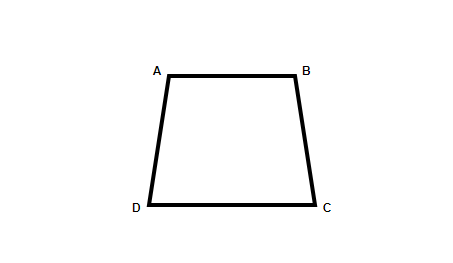Solution:

We already know, In a Trapezium, two opposite sides are parallel to each other, here, AB is parallel to CD

The Interior angles formed by two parallel lines have a sum of 180°(Property of parallel lines)

Therefore, we can write, ∠A + ∠D = 180°

100° + ∠D = 180°

∠D = 80°

Similarly, ∠B+ ∠C = 180°

∠B + 80° = 180°

∠B = 100°

Question 5: In the figure below, the interior angles of the quadrilateral are given as,

∠ABC = 50°, ∠BAD = 20°, ∠BCD = 10°

Find the value of the exterior angle ∠ADC?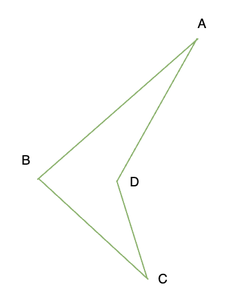Solution:

In a quadrilateral, the sum of all the interior angles is 360°

50° + 20° + 10° + ∠ADC = 360°

The angle that came out is the interior angle, the sum of interior angle and the exterior angle will be 360,

Exterior angle ∠ADC = 360 – 280 = 80°

Question 6: In the given parallelogram ABCD, the value of an interior angle is 60°. Find the values of all other angles.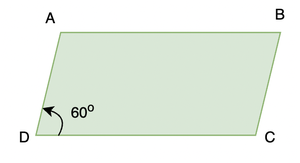Solution:

The value of ∠D is given to be 60°. We need to find other angles.

We know that sum of adjacent angles in a parallelogram is 180°. So let the value of ∠A be x.

x + 60° =180°

x = 120°

∠A = 120°

We also know that opposite angles in a parallelogram are equal.

So,

∠A = ∠C and ∠D = ∠B

So, ∠A = 120°, ∠B = 60°, ∠C = 120° and ∠D = 60°

Question 7: In the given quadrilateral, ∠A = 2x°, ∠B = x°, ∠C = 90° and ∠D = 3x°. Find the value of the largest angle.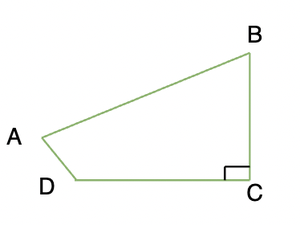Solution:

We know that by the angle sum property, sum of all the interior angles of a quadrilateral is 360o

So, ∠A + ∠B + ∠C + ∠D = 360°

Given that ∠C = 90°

Let’s plug in the rest of the values given,

2x + x + 90 + 3x = 360

⇒ 6x = 360 – 90

⇒ 6x = 270

⇒ x = 45°

So, the largest angle is ∠D = 3x = 3(45) = 135°

My Personal Notes arrow_drop_up